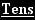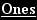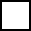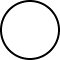Create a new printableMath Worksheets

Sample - Click above to make a new math worksheet (PDF).
 Name _____________________________Date ___________________
Write the sum.
1.3 7 + 1
2.7 7 + 2
3.3 5 + 3
4.2 + 8 2

Fill in the missing numbers to complete the addition fact.
5.+ 6 7 6 8
6.7 0 + 22
7.6 3 +6 8
8.+ 2 2 2 6

 Key #2
 aKey #2
Write the sum.
9.7 0 + 1 0
10.2 0 + 5 3
11.1 1 + 5 3
12.2 9 + 1 0

Fill in the missing numbers to complete the addition fact.
13.7 0 + 1 99
14.8 6 + 19 8
15.5 7 +2 9 9
16.3 + 8 0 9 3

Write the sum.
 17 17  +  71  =  _____
 18 27  +  70  =  _____
 19 50  +  49  =  _____
 20 30  +  69  =  _____
 21 68  +  11  =  _____
 22 28  +  61  =  _____

 Key #2
 aKey #2
Fill in the missing numbers to complete the addition fact.
23.
 30 +74
24.
 28 +78
25.+ 13 97
26.+ 21 88
27.+ 39 59

Write an addition sentence to solve each word problem.
28.
 Sarah counted 39 oak leaves and 20 elm leaves in her backyard. How many leaves were there altogether? ____________________ leaves
29.
 Anthony found 52 pennies. Kaylee found 17 pennies. How many pennies did they find in all? ____________________ pennies

Write an addition sentence to solve each word problem.
30.
 Courtney has 33 marbles. Kevin has 20 marbles. How many marbles do they have in all? ____________________ marbles
31.
 Caleb and his family traveled 72 miles to his uncle's house and 24 miles back home. How many miles did they travel in all? ____________________ miles